## A 50 g copper calorimeter contains 250 g of water at 20 C. How much steam be condensed into the water to make the final temperature of the s

Question

A 50 g copper calorimeter contains 250 g of water at 20 C. How much steam be condensed into the water to make the final temperature of the system 50 C. ( specific heat water= 4200 J/Kg C , specific heat copper= 390 J/Kg C

in progress 0
6 months 2021-07-20T03:01:58+00:00 1 Answers 18 views 0

Approximately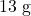of steam at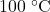(assuming that the boiling point of water in this experiment is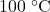.)

Explanation:

Latent heat of condensation/evaporation of water: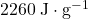.

Both mass values in this question are given in grams. Hence, convert the specific heat values from this question to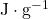.

Specific heat of water: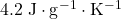.

Specific heat of copper: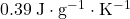.

The temperature of this calorimeter and the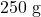of water that it initially contains increased from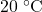to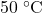. Calculate the amount of energy that would be absorbed: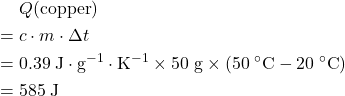.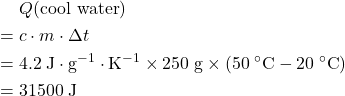.

Hence, it would take an extra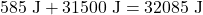of energy to increase the temperature of the calorimeter and theof water that it initially contains fromto.

Assume that it would take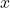grams of steam atensure that the equilibrium temperature of the system is.

In other words,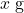of steam atwould need to release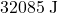as it condenses (releases latent heat) and cools down to.

Latent heat of condensation fromof steam: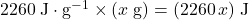.

Energy released when that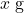of water from the steam cools down fromto: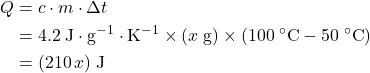.

These two parts of energy should add up to. That would be exactly what it would take to raise the temperature of the calorimeter and the water that it initially contains fromto.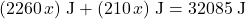.

Solve for: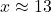.

Hence, it would take approximatelyof steam atfor the equilibrium temperature of the system to be.